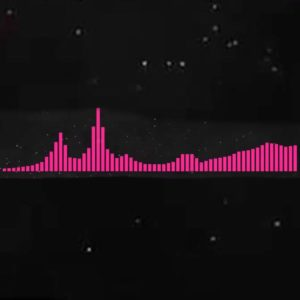# Whistle (Easy)

16 September 2020
2
• ##### TYPE
0
(0)

6 j h f h f 8 f d s d f
6 j h f h f 8 5
a a a a s s p o s s p o s d d 5
a a a a s s p o s s p o s d d 5
s s j j h h f f d s f h f 5
a a a a s s p o s s p o 8 d a s
6 0 j h f h f 8 w f d s d f
6 0 j h f h f 8 w f d s d f

Rate This Music Sheet:

Average rating 0 / 5. Vote count: 0

No votes so far! Be the first to rate this music sheet.

Thank you for rating this song!

If you have any specific feedback about how to improve this music sheet, please submit this in the box below.

Whistle (Easy) is a song by Flo Rida. Use your computer keyboard to play Whistle (Easy) music sheet on Virtual Piano. This is an Easy song and requires practice. The song Whistle (Easy) is classified in the genres: USA, Pop on Virtual Piano. You can also find other similar songs using Hip hop.

## Other Songs By Flo Rida

•aaa a [s6] s p o [s4] s p o [s8] d d 5 a a aa [s6] s p o [s4] s p o [s8] d d 5| s s [j6] j h h [f4] f d s [f8] h f 5 aaa a [s6] s p o [s4] s p o 8| | [d5] a s 6| | [j4] h f hf8| | [f5] d s df6| | [j4] h f hf8| | [f5] d s df6|| ps4ss s s 8| oos[s5]ss d s 6| oos4ss s os8s os ps5pssd s 6 oss f4ss s o8 osssf5ss s p6 sss ss4as s p8 ssssf5ss s o6 sss s [s4]ss s s [s8]ss s s [s5]ssss o6 sss s [s4]ss s o8 sss ss[s5]saaa a [s6] s p o [s4] s p o [s8] d d 5 a a aa [s6] s p o [s4] s p o [s8] d d 5| s s [j6] j h h [f4] f d s [f8] h f 5 aaa a [s6] s p o [s4] s p o 8| | [d5] a s 6| | s [s6] sss d 4| spsp[s8]psps o 5| sas [s6] s s d 4 ssffff[f8] f f f [f5] f d s 6 o| ss[s4] s s d [s8]| s| [a5]| p| 6 s| ss[s4] s s d [s8]| s| [d5] aaa a [s6] s p o [s4] s p o [s8] d d 5 a a aa[s6] s p o [s4] s p o
Level: 4
Length: 01:46
Easy

## Related

•[8f]|[9d]|[0a]| [8f]|[9d]|[0a]| [8f]|[9d]|[0a] [wa]|[9d]|[0u]| [8f]|[9d]|[0a] p o [8f]|[9d]|[0a] [8f]|[9d]|[0a] p o p [wa]|[0d]|[0u] | [8f]|[9d]|[0a] p o [8f]|[9d]|[0s] a [8f]|[9d]|[0a] p o p [wa]|[9d]|[0u] | a o 8 9 0 p o [8p] o 9 0 u u [8a] a a [9p] p p [o0] u u o o p [wa]|[9d]|[0a] | a o 8 9 0 p o [8p] o 9 0 u u [8a] a a [9p] p [o0] u u o o p [wa]|[9d]|[0a] | p [wa]|[9d]|[0f]
Level: 4
Length: 01:00
Easy

#### Billie Eilish

•[ts] u o u [ep] t u t [qi] e t e [wo] r y r
t u [ov] [uv] [ev] t u [tv]C [qx] [ex]C [tv] [ex] [wz] r [yl] [rz] [tx] [ul] [oh] u
e t u t q e t e w r y r
t u [ov] [ub] [ev] t u [tx]C [qv] [ec]x [tz] [eJ] [wj] r [yd] [rh] [th] [uz] [ol] u e t u t q e t e w r y r
[th] [uf] [og] [ud] [ef] [ts] [ud] [tp]i [qo] [ei] [to] [ej] [wh] r y r
[th] [uf] [og] [ud] [ef] [ts] [ud] [tp]i [qo] [ei] [to] [ej] [wh] r y r

Level: 4
Easy

#### Dan Salvato

•i i i o O s P P O P s P O i i i o O i s P O P s P O g g g h H c c c Z l | l J l v v v x V c g g g h H c c c Z l | H J l J l J H h H g g g g h H c c c Z l | l J l v v v x V c g g g h H c c c Z l | H J l J l J H h H g s O i t w q
Level: 3
Easy